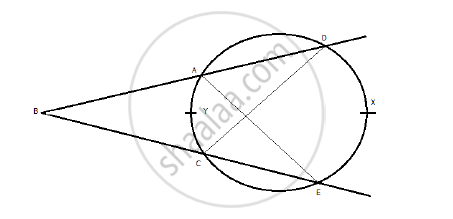# In the following figure, if m(are DXE) = 90° and m(are AYC) = 30°. Find ∠DBE. - Geometry

In the following figure, if m(are DXE) = 90° and m(are AYC) = 30°. Find ∠DBE.#### SolutionBy inscribed angle theorem,

m∠AEB=(1/2) × m∠AYC=(1/2) × 30o = 15...(1)

m∠EAD=(1/2) × m∠DXE=(1/2) × 90o = 45o ...(2)

⇒ m∠DBE + 15o = 45o

⇒ m∠DBE = 45o - 15o = 30o

Concept: Areas of Sector and Segment of a Circle
Is there an error in this question or solution?
2014-2015 (March) Set B

Share